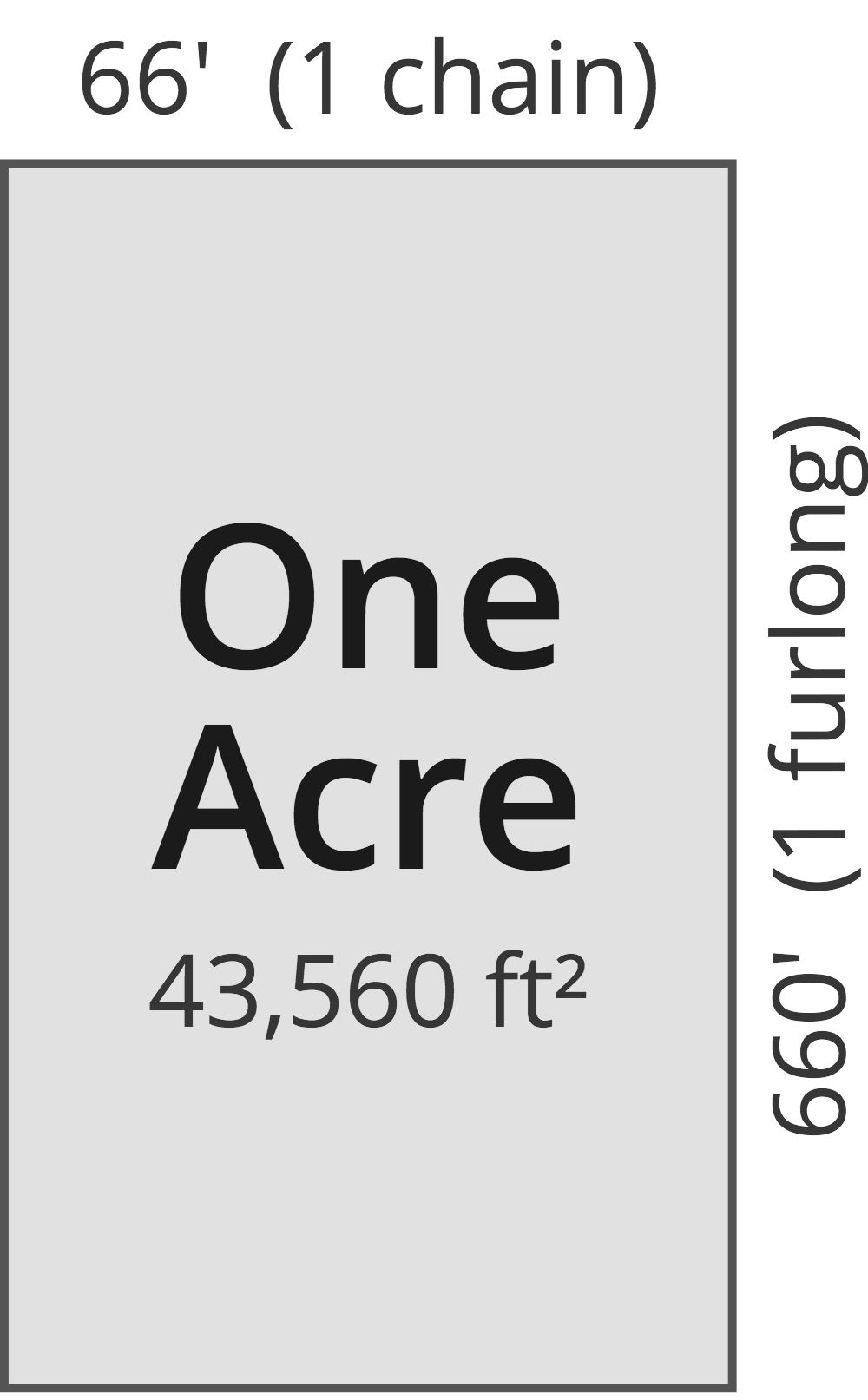# Square Centimeters to Acres Converter

Enter the area in square centimeters below to get the value converted to acres.

Results in Acres:1 sq cm = 2.4711E-8 ac
Hint: use a scientific notation calculator to convert E notation to decimal

Do you want to convert acres to square centimeters?

## How to Convert Square Centimeters to Acres

To convert a measurement in square centimeters to a measurement in acres, multiply the area by the following conversion ratio: 2.4711E-8 acres/square centimeter.

Since one square centimeter is equal to 2.4711E-8 acres, you can use this simple formula to convert:

acres = square centimeters × 2.4711E-8

The area in acres is equal to the area in square centimeters multiplied by 2.4711E-8.

For example, here's how to convert 50,000,000 square centimeters to acres using the formula above.
acres = (50,000,000 sq cm × 2.4711E-8) = 1.235527 ac

## What Is a Square Centimeter?

One square centimeter is equivalent to the area of a square with 1 centimeter long sides. One square centimeter is equal to 0.155 square inches or 1/10,000 of a square meter.

The square centimeter, or square centimetre, is a multiple of the square meter, which is the SI derived unit for area. In the metric system, "centi" is the prefix for hundredths, or 10-2. A square centimeter is sometimes also referred to as a square cm. Square centimeters can be abbreviated as sq cm, and are also sometimes abbreviated as cm². For example, 1 square centimeter can be written as 1 sq cm or 1 cm².

## What Is an Acre?

One acre is defined as the area equal to a space that is one chain (66 ft) by one furlong (660 ft), or 10 square chains. That's equal to 43,560 square feet or 1/640 of a square mile for those unfamiliar with those units of measure.The acre is a US customary and imperial unit of area. Acres can be abbreviated as ac; for example, 1 acre can be written as 1 ac.

You can use an acreage calculator to measure the area of a plot of land in acres by locating the boundaries on a map.

## Square Centimeter to Acre Conversion Table

Table showing various square centimeter measurements converted to acres.
Square Centimeters Acres
1 sq cm 0.000000024711 ac
2 sq cm 0.000000049421 ac
3 sq cm 0.000000074132 ac
4 sq cm 0.000000098842 ac
5 sq cm 0.00000012355 ac
6 sq cm 0.00000014826 ac
7 sq cm 0.00000017297 ac
8 sq cm 0.00000019768 ac
9 sq cm 0.00000022239 ac
10 sq cm 0.00000024711 ac
100 sq cm 0.0000024711 ac
1,000 sq cm 0.000024711 ac
10,000 sq cm 0.000247 ac
100,000 sq cm 0.002471 ac
1,000,000 sq cm 0.024711 ac
10,000,000 sq cm 0.247105 ac
100,000,000 sq cm 2.4711 ac

## References

1. National Institute of Standards and Technology, Specifications, Tolerances, and Other Technical Requirements for Weighing and Measuring Devices, Handbook 44 - 2019 Edition, https://nvlpubs.nist.gov/nistpubs/hb/2019/NIST.HB.44-2019.pdf